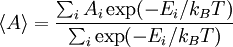# Boltzmann average

(diff) ← Older revision | Latest revision (diff) | Newer revision → (diff)
The Boltzmann average (sometimes known as the thermal average) for a given quantity or observable, let us say$A$, is given by$\langle A \rangle = \frac{\sum_i A_i \exp(-E_i/k_B T)}{\sum_i\exp(-E_i/k_B T)}$
where$k_B$ is the Boltzmann constant, and T is the temperature. This provides the expected value of the property in question at a given temperature.